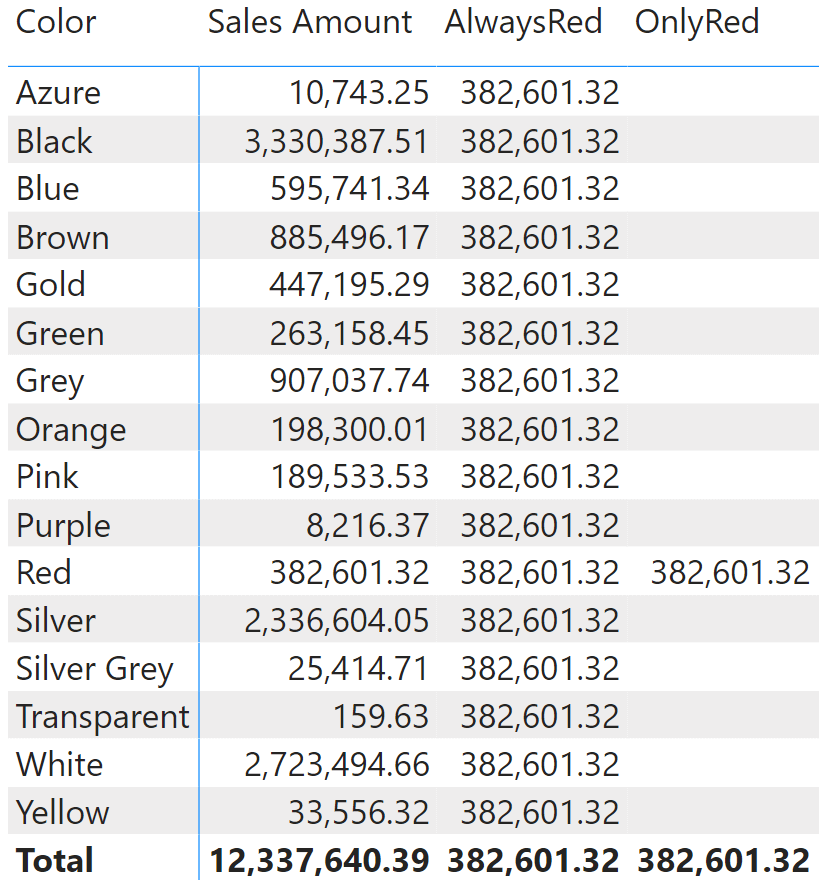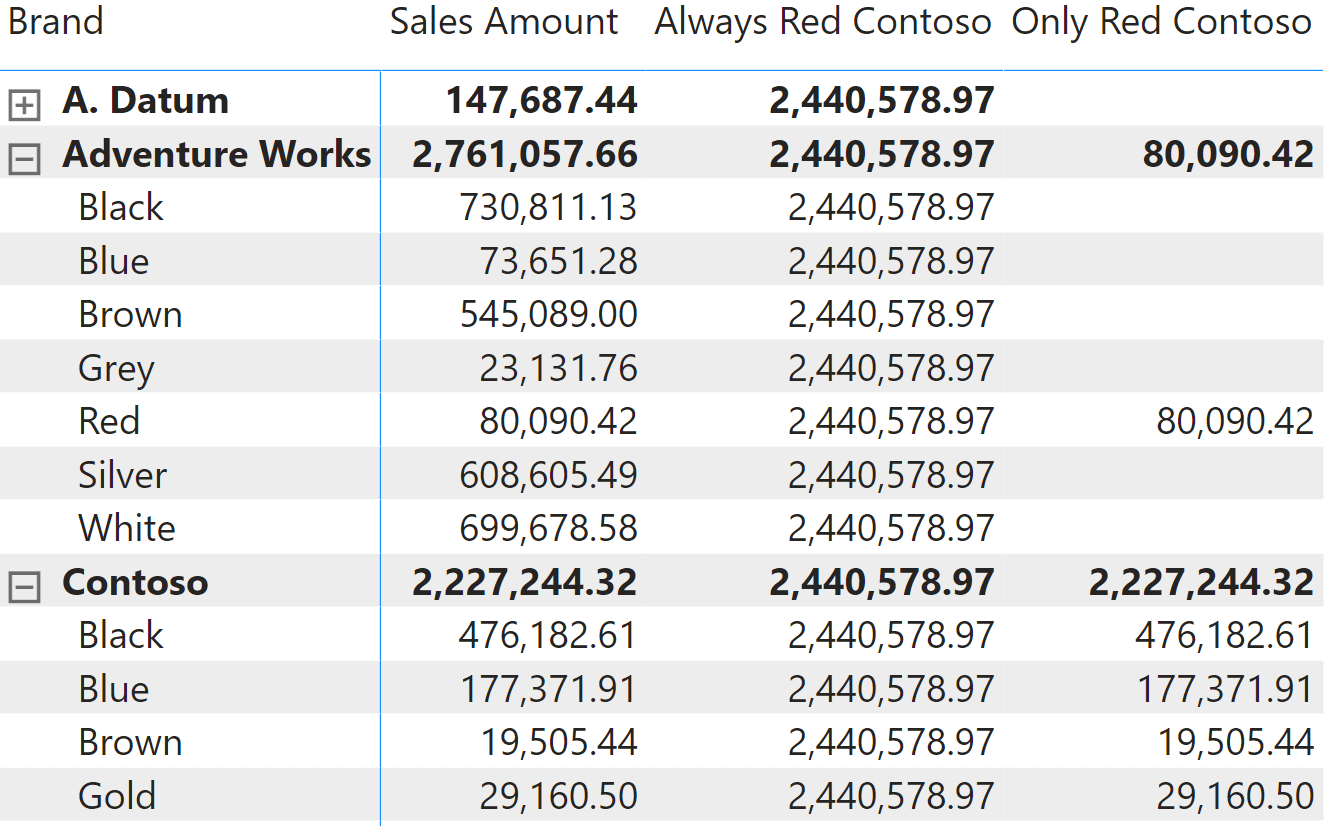KEEPFILTERS is a CALCULATE modifier used to change the way CALCULATE merges new filters with the outer filter context. Indeed, the default behavior of CALCULATE is to override existing filters. By using KEEPFILTERS you ask CALCULATE to add the new filter to the outer filter context, instead of overriding the outer filter.

## Single column filters

Let us start by looking at the effect of using KEEPFILTERS in a simple measure that uses CALCULATE with a single column filter. AlwaysRed shows the sales amount of red products, by forcing the Product[Color] to be read while the value of Sales Amount is being computed:

Measure in Sales table
```AlwaysRed :=
CALCULATE (
[Sales Amount],
'Product'[Color] = "Red"
)
```

Bear in mind that the compact syntax of CALCULATE is translated into a longer syntax, because filters in CALCULATE are always tables. If you are not familiar with this concept, you may find more information by reading Introducing CALCULATE in DAX. The following is an equivalent formulation of the same measure:

Measure in Sales table
```AlwaysRed :=
CALCULATE (
[Sales Amount],
FILTER (
ALL ( 'Product'[Color] ),
'Product'[Color] = "Red"
)
)
```

The presence of ALL – which is explicit in this latter version while being implicit in the earlier version – means that the existence of an outer filter over Product[Color] is ignored by CALCULATE. This forces the color to be red regardless of any outer filter.

You can change this behavior by using KEEPFILTERS like in the OnlyRed measure. The OnlyRed measure is identical to AlwaysRed, except for the use of KEEPFILTERS around the filter:

Measure in Sales table
```OnlyRed :=
CALCULATE (
[Sales Amount],
KEEPFILTERS ( 'Product'[Color] = "Red" )
)
```

You can see that the two measures behave differently regarding the outer filter. AlwaysRed always returns the sales volume of red products, as it replaces the outer filter with the red color. OnlyRed on the other hand returns a value only when red is already selected in the outer filter.You can obtain this behavior by using different techniques, that you can find in many articles and blogs written prior to the introduction of KEEPFILTERS in DAX. By using VALUES as an additional filter in the compact syntax, or by replacing ALL with VALUES in the extended syntax, you achieve the same goal:

Measure in Sales table
```OnlyRed Values :=
CALCULATE (
[Sales Amount],
Product[Color] = "Red",
VALUES ( 'Product'[Color] )
)
```
Measure in Sales table
```OnlyRed Values Ext :=
CALCULATE (
[Sales Amount],
FILTER (
VALUES ( 'Product'[Color] ),
Product[Color] = "Red"
)
)
```

As you can see, the three measures behave the same way, even though the version using KEEPFILTERS is usually more efficient.## Multiple column filters

KEEPFILTERS can also be used with conditions involving multiple columns. For example, if you want to test both the color and the brand in the same condition, you can author the following measure:

Measure in Sales table
```AlwaysRedContoso :=
CALCULATE (
[Sales Amount],
OR (
'Product'[Color] = "Red",
'Product'[Brand] = "Contoso"
)
)
```

When used in a matrix, it shows the same number on each row. Indeed, both the filter on the brand and the filter on the color were replaced.Below, we see a scenario where KEEPFILTERS merges the filter on both columns with the outer filter context:

Measure in Sales table
```OnlyRedContoso :=
CALCULATE (
[Sales Amount],
KEEPFILTERS (
OR (
'Product'[Color] = "Red",
'Product'[Brand] = "Contoso"
)
)
)
```

This results in blanks everywhere except on the intersection of Contoso and Red.As was the case with the single column scenario, you can obtain the same behavior by using multiple filters in CALCULATE. Alas, in the multiple column scenario you cannot leverage VALUES, because VALUES works with a single column only. However, you can use SUMMARIZE to obtain the desired effect, both with the compact and with the extended syntax:

Measure in Sales table
```OnlyRedContoso SUMMARIZE :=
CALCULATE (
[Sales Amount],
OR (
'Product'[Color] = "Red",
'Product'[Brand] = "Contoso"
),
SUMMARIZE ( 'Product', 'Product'[Color], 'Product'[Brand] )
)
```
Measure in Sales table
```OnlyRedContoso SUMMARIZE Ext :=
CALCULATE (
[Sales Amount],
FILTER (
SUMMARIZE ( 'Product', 'Product'[Color], 'Product'[Brand] ),
OR (
'Product'[Color] = "Red",
'Product'[Brand] = "Contoso"
)
)
)
```

## KEEPFILTERS in iterators

KEEPFILTERS can also be used with iterators. In this case, KEEPFILTERS is still a CALCULATE modifier although there seems to be no CALCULATE. We see this in the following example:

Measure in Sales table
```Monthly Average :=
AVERAGEX (
KEEPFILTERS ( VALUES ( 'Date'[Month] ) ),
[Sales Amount]
)
```

Here, KEEPFILTERS is used to modify the semantics of the context transition induced by the Sales Amount measure reference. Although that aspect is too advanced for this introductory article, it is a nice transition to a more advanced piece. If you want to better understand when and how to use KEEPFILTERS with iterators, you can find more information here: When to use KEEPFILTERS over iterators.

KEEPFILTERSCALCULATE modifier

Changes the CALCULATE and CALCULATETABLE function filtering semantics.

`KEEPFILTERS ( <Expression> )`

CALCULATEContext transition

Evaluates an expression in a context modified by filters.

`CALCULATE ( <Expression> [, <Filter> [, <Filter> [, … ] ] ] )`

ALLCALCULATE modifier

Returns all the rows in a table, or all the values in a column, ignoring any filters that might have been applied.

`ALL ( [<TableNameOrColumnName>] [, <ColumnName> [, <ColumnName> [, … ] ] ] )`

VALUESWhen a column name is given, returns a single-column table of unique values. When a table name is given, returns a table with the same columns and all the rows of the table (including duplicates) with the additional blank row caused by an invalid relationship if present.

`VALUES ( <TableNameOrColumnName> )`

SUMMARIZECreates a summary of the input table grouped by the specified columns.

`SUMMARIZE ( <Table> [, <GroupBy_ColumnName> [, [<Name>] [, [<Expression>] [, <GroupBy_ColumnName> [, [<Name>] [, [<Expression>] [, … ] ] ] ] ] ] ] )`